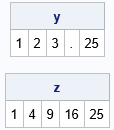Converting a program from one language to another can be a challenge. Even if the languages share many features, there is often syntax that is valid in one language that is not valid in another. Recently, a SAS programmer was converting a program from R to SAS IML. He reached out to me because his direct translation from R to IML failed to work. Fortunately, with a little knowledge of the way that the languages differ, I was able to write a user-defined function that duplicated a language feature in R that gives an error in SAS (and other languages).

### Indexing beyond the bounds of an array in R

The issue involved specifying an index that is greater than the length of an array. For example, if you define an array that has three elements, what happens if you attempt to access the fifth element of the array?

In most languages, it is an error to index beyond the bounds of an array. In C, Java, SAS, and many other languages, your program will throw an exception, display an error, or crash if you attempt to index outside of the valid bounds. Not so in R. The R language does not consider this an error. R handles the issue as follows:

• If you are reading a value from an array, a missing value (NA) is returned.
• If you are assigning a value into an array, the array is resized. The assignment operation is then performed on the larger array.

For example, the following R program shows the results when you use the fifth element of a three-element array:

```# R language y = 1:3 # Ex1: allocate a 3-element vector y = 25; # grow the array to 5 elements; perform the assignment u = 1:3 # Ex2: allocate a 3-element vector t = u # ask for the value of the 5th element   print(y) print(t)```
```> print(y)  1 2 3 NA 25 > print(t)  NA```

The output shows the results in R. In the first example (assignment), the y vector is reallocated to a five-element vector. A missing value (NA) is used for the value of the new elements. The assignment to the fifth element then proceeds. In the second example, a missing value is returned from the query, u.

This feature is sometimes used by R programmers to grow the length of an array dynamically. For example, each iteration of the following loop dynamically increases the length of an array. At the beginning of the loop, the vector was empty; at the end of the loop, the vector has five elements:

```# R language z = numeric(0) # allocate a 0-length vector for (i in 1:5) { value = i^2; z[i] = value # grow the array and perform the assignment } print(z)```
```> print(z)  1 4 9 16 25```

I generally avoid resizing arrays inside a loop because it can be inefficient. However, sometimes you do not know in advance the final length of the result vector. In that case, this dynamic resizing is convenient.

### Growing vectors in SAS IML

I briefly considered rewriting the original R program so that it does not use this feature that dynamically grows arrays. However, I was not familiar with the underlying algorithm, so I decided it would be easier to write an IML subroutine that emulates the R behavior. The program only used the "assignment version" of this feature (indexing on the left of an equal sign), so I wrote the following IML subroutine:

```/* duplicate an R feature where an array is expanded if you assign an index that is larger than the length of the array. */ proc iml; /* Input: x is a row vector of length n. Output: x[k] = value Effect: If k > n, then reallocate the length of the vector, pad with missing values, and perform the assignment. */ start AssignValue(x, k, value); n = ncol(x); if k <= n then x[k] = value; else do; y = j(1, k, .); /* reallocate x to a larger size; pad with missing */ if n > 0 then y[1:n] = x; /* copy existing data */ y[k] = value; /* assign the kth element */ x = y; /* overwrite elements of x */ end; finish;   /* test the subroutine */ y = 1:3; * allocate a 3-element vector; call AssignValue(y, 5, 25); * grow the array to 5 elements... perform the assignment; print y;   z = {}; * allocate a 0-length vector; do i = 1 to 5; value = i##2; call AssignValue(z, i, value); * grow the array and perform the assignment; end; print z;```Success! The AssignValue subroutine takes an existing vector and assigns the kth element of the vector, growing the vector if necessary. With the help of this subroutine, the conversion of the algorithm from the R language to SAS IML went smoothly.

### Summary

Converting a program from one language to another can be a challenge, especially if the program uses features of the first language that are not supported in the second language. In this situation, you have two choices: rewrite the program so that it doesn't use the language feature or emulate it in the second language. If you do not have technical knowledge of the algorithm, it might be easier to emulate the language feature in the second language. Note that you don't have to emulate all details of the feature, only the ones that are used in the program.

This article discusses these ideas in the context of converting a program from R to SAS IML. The original program used a feature of R that dynamically grows vectors when an assignment statement uses an index that is out of bounds. By writing a SAS IML module that emulates this behavior, the R program was successfully converted.

Share